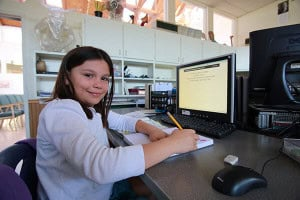# MathThe Heron Basics Mathematics Seminar Program for grades K through 5 provides a high level of communication in math and produces a solid understanding of the key math concepts.  Lessons are given seminar-style, providing lively classroom interaction, followed by mathematics drilling.

Emphasis is on fully understanding the numeral system and place value, defining all terminology, learning to write work out neatly and check it for accuracy, and mastering all arithmetic actions. The common areas of “math quicksand” (such as fractions), which so many math series skirt past in math programs, are fully tackled and cleared up.  This program adds full fluency with numbers to the literacy produced in other Heron Basics programs.

The Math Facts on the Computer Program is a supporting part of the Heron Basics Mathematics Program that teaches a student to know the facts of arithmetic on an instant response basis. This program uses a computer to present math facts to the student requiring the student to enter the correct answer. It requires regular drilling of 15-30 minutes daily, and results in students having the tool of instant response ability while doing their other mathematics – arithmetic just becomes an “invisible” skill so students can put their energy into getting on with actual mathematics learning. There is also a mental math section that gives students practice in rapidly figuring out problems “in their heads.”

#### READY TO FIND OUT MORE?

Fill out the form below to receive:

• Results
• Curriculum
• Tuition
• School Calendar